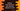# Python program to find the maximum and minimum element in a list## Python program to find the maximum and minimum element in a list :

In this tutorial, we will learn how to find the maximum and minimum number in a python list. Python list can hold items of any data types. All items are separated by a comma _and placed inside a _square bracket. We can access any item by using its index. The index starts at 0. The index for the first element is 0, the index of the second element is 1 etc.

In this blog post, I will show you how to find the maximum and minimum number in a list using a loop. All the numbers will be entered by the user. The user will enter the list items one by one and our program will read them.

First, we will ask the user to enter the total number count. Then using a for loop, we will read each number and append them to the list. Finally, again using one more for loop, we will calculate the maximum and minimum number and print out the result.

Let’s take a look into the program first :

``````#1
my_list = []

#2
count = int(input("How many numbers you want to add : "))

#3
for i in range(1,count+1):
my_list.append(int(input("Enter number {} : ".format(i))))

#4
print("Input Numbers : ")
print(my_list)

#5
min = my_list
max = my_list

#6
for no in my_list:
if no < min : min = no elif no > max :
max = no

#7
print("Minimum number : {}, Maximum number : {}".format(min,max))``````

The source code is available here.

### Explanation :

The commented numbers in the above program denote the step number below :

1. Create one empty list mylist. We are using one _empty square bracket to create the empty list. This is a list without any items.
2. Get the total number of elements the user is going to enter and save it in the count variable. This is required because we will ask the user to enter each number one by one. If the value of count is 4, the user will have to enter four numbers to add to the list.
3. Using a_ for loop, get the numbers and append it to the list _mylist. For appending a number, _append _method is used. This method takes the value we are adding as the parameter. For reading the value, we are using the _input _method. This method will read the value from the user. The return value of this method is of _string _type. Wrapping it as int()_ will convert the entered value to an integer.
4. Print the list to the user.
5. Create two variables to hold the minimum and maximum number. We are assigning the first element of the list to both of these variables first. We will update these variables on the next step. On this step, we are assuming that the minimum and maximum value of the list is equal to the first element of the list. We will compare this value with all other elements of the list one by one and update them if required.
6. Run one for the loop on the list again. For each number, check if it is less than the minimum number. If yes, assign the minimum value holding variable to this number. Similarly, update the maximum value if the number is more than the current maximum.
7. After the list is completed reading, print out both maximum and minimum numbers.